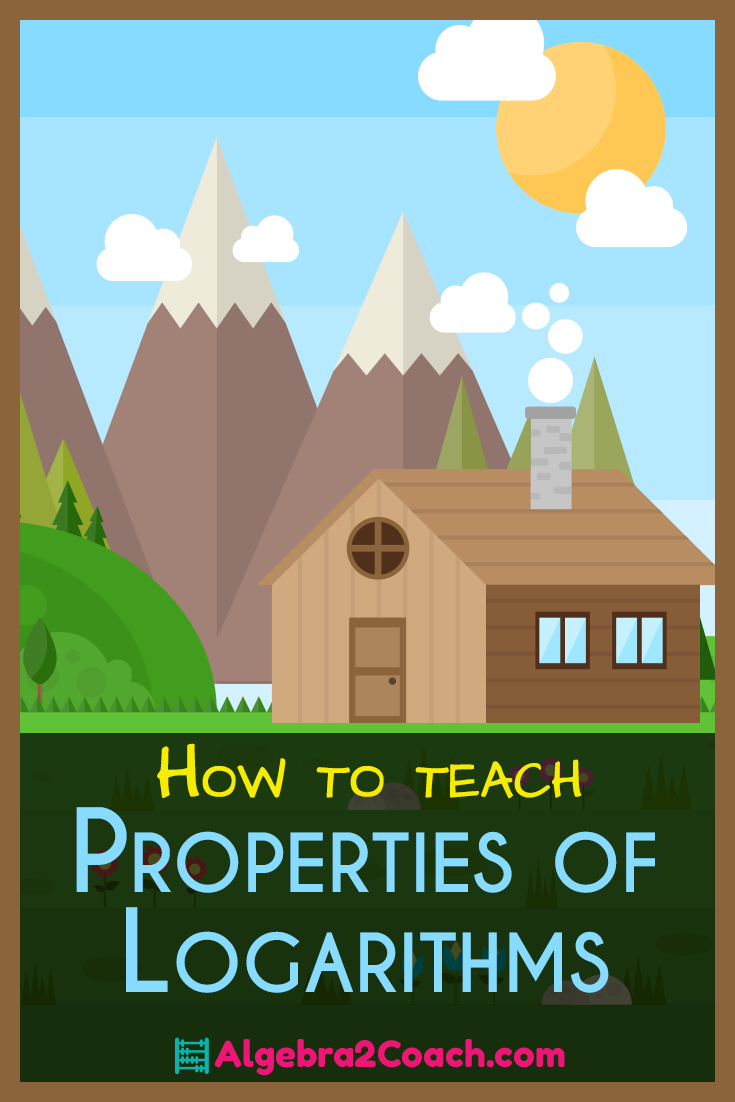# Properties of Logarithms

 Would You Rather Listen to the Lesson?## Change of Base Formula

Your calculator will only do Base 10 (log) and base e (ln). In most cases this is sufficient for real world problems. However, if another base is needed we have to be able to adjust! The best example you can use in class is a CLOCK! A clock doesn't use either of these bases. Then ask them this high level question.
"Make a list of 5 real world situations where we would use a base other than Base 10 (log) and base e (ln)."
That will bring them to a questions...
"So how do we do problems where we need to use these other bases?"

Now you can explain and teach them how to use the Change of Base Formula.
Take the log of the problem divided by the log of the base.

loga x = ( logb x ) / ( logb a )

The base that you use doesn't matter, only that you use the same base for both the numerator and the denominator.
loga x = ( log x ) / ( log a ) = ( ln x ) / ( ln a )
Example: log5 8 = ( ln 8 ) / ( ln 5 )
Properties of Logarithms (and Exponents)
Exponents and Logarithms share the same properties. It may be a good idea to review the properties of exponents prior to introducing logs. Here are the properties...

## Multiplication Rule

They have it pounded into their heads. Same base different exponent being multiplied you add the exponents.
Same applies for logs. When you multiply, you're going to add the logarithms.

The log of a product is the sum of the logs.

loga xy = loga x + loga y

## Division Rule

They then use common sense to remember that if when you multiply you add the exponents then when you divide two values with the same base you must subtract the exponents. Therefore, the rule for division of logs is to subtract the logarithms.

The log of a quotient is the difference of the logs.

loga (x/y) = loga x - loga y

## Raising to a Power Rule

When you raise same base with any exponents to a power, the rule is that you multiply the exponents together. In this case, one of the exponents is the log, and the other exponent is the power you're raising the quantity to.
The exponent on the argument is the multiplied by the log. (You could also say it becomes the coefficient of the log)

loga xr = r * loga x

## Summary - Properties of Logarithms

• The log of a product is the sum of the logs
• The sum of the logs is the log of the products
• The log of a quotient is the difference of the logs
• The difference of the logs is the log of the quotient
• The exponent on the argument is the coefficient of the log
• The coefficient of the log is the exponent on the argument

## Most Common Mistakes

• The log of a sum is NOT the sum of the logs. The sum of the logs is the log of the product. The log of a sum cannot be simplified.
loga (x + y) ≠ loga x + loga y

• The log of a difference is NOT the difference of the logs. The difference of the logs is the log of the quotient. The log of a difference cannot be simplified.
loga (x - y) ≠ loga x - loga y
• An exponent on the log is NOT the coefficient of the log. Only when the argument is raised to a power can the exponent be turned into the coefficient. When the entire logarithm is raised to a power, then it can not be simplified.
(loga x)r ≠ r * loga x

• The log of a quotient is not the quotient of the logs. The quotient of the logs is from the change of base formula. The log of a quotient is the difference of the logs.
loga (x / y) ≠ ( loga x ) / ( loga y )

Do you have a Sub on Log Day? Here you go...

## Here is Your Free Content for This Lesson!

### Properties of Logarithms Worksheet, Word Docs & PowerPoints

To gain access to our editable content Join the Algebra 2 Teacher Community!
Here you will find hundreds of lessons, a community of teachers for support, and materials that are always up to date with the latest standards.

### Properties of Logarithms - PDFs

8-4 Guided Notes Teacher Edition - Properties of Logarithms (Members Only)

8-4 Lesson Plan - Properties of Logarithms (Members Only)

8-4 Online Activities - Properties of Logarithms (Members Only)

### Don't forget to Pin this lesson!Posted in Algebra 2 Worksheets, Blog and tagged , , , , .

1.Head’s? Their head’s what? Shouldn’t there be no apostrophe there? Apols for nitpicking

2.Algebra2Coach.com Team

Good catch, thank you. Fixed. 🙂

3.Phung N.

4.